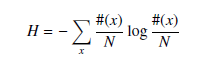# 1988. Strange String Manipulation

A linear congruential generator produces a series R(・) of pseudo-random numbers by the following formulas:

R(0) = S, R(i) = (A * R(i - 1) + C) mod M (for i = 1, 2, . . .),

where S , A, C, and M are all parameters. In this problem, 0 <= S,A,C <= 15 and M = 256.

Now suppose we have some input string I(・), where each character in the string is an integer between 0 and (M - 1). Then, using the pseudo-random number series R(・), we obtain another string O(・) as the output by the following formula:

O(i) = (I(i) + R(i)) mod M (for i = 1, 2, . . .),

Your task is to write a program that shows the parameters S , A, and C such that the information entropy of the output string O(・) is minimized. Here, the information entropy H is given by the following formula:where N is the length of the string and #(x) is the number of occurences of the alphabet x.

### 输入格式

The input has the following format:

N

I(1) I(2) . . . I(N)

N does not exceed 256.

### 输出格式

Print in a line the values of the three parameters S , A, and C separated by a single space. If more than one solution gives the same minimum entropy, choose the solution with the smallest S , A, and then C.

### 样例

Input
5
5 4 3 2 1
/*
5
7 7 7 7 7
10
186 8 42 24 154 40 10 56 122 72
*/

Output
0 1 1
/*
0 0 0
8 7 14
*/


25 人解决，35 人已尝试。

34 份提交通过，共有 135 份提交。

5.0 EMB 奖励。